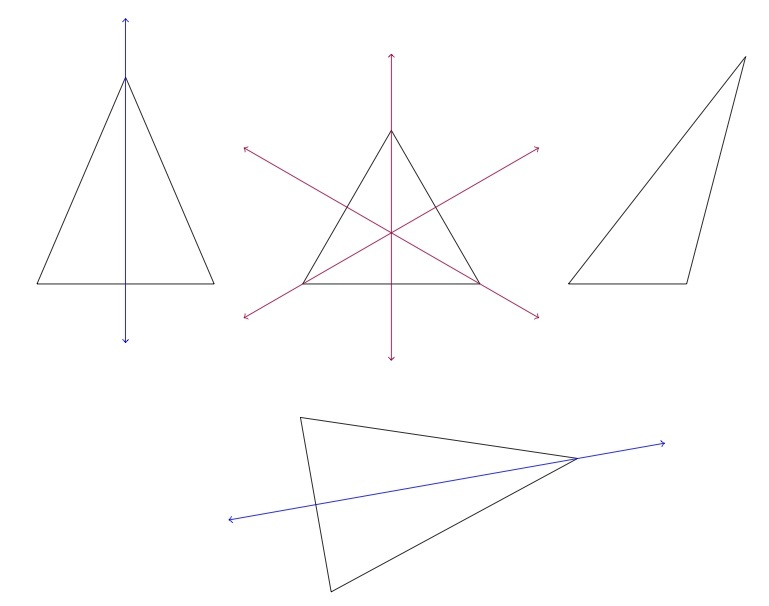# Lines of symmetry for triangles

Alignments to Content Standards: 4.G.A.3

Below are pictures of four triangles with given side lengths:For each triangle, find and draw all lines of symmetry.

## IM Commentary

The division of triangles into scalene, isosceles, and equilateral can be thought of in terms of lines of symmetry. A scalene triangle is a triangle with no lines of symmetry while an isosceles triangle has at least one line of symmetry and an equilateral triangle has three lines of symmetry. This activity provides students an opportunity to recognize these distinguishing features of the different types of triangles before the technical language has been introduced. For finding the lines of symmetry, cut-out models of the four triangles would be helpful so that the students can fold them to find the lines.

This task is intended for instruction, providing the students with a chance to experiment with physical models of triangles, gaining spatial intuition by executing reflections. A word has been added at the end of the solution about why there are not other lines of symmetries for these triangles: this has been inserted in case this topic comes up in a class discussion but the focus should be on identifying the proper lines of symmetry.

## Solution

The lines of symmetry for the four triangles are indicated in the picture below:A line of symmetry for a triangle must go through one vertex. The two sides meeting at that vertex must be the same length in order for there to be a line of symmetry. When the two sides meeting at a vertex do have the same length, the line of symmetry through that vertex passes through the midpoint of the opposite side. For the triangle with side lengths 4,4,3 the only possibility is to fold so the two sides of length 4 align, so the line of symmetry goes through the vertex where those two sides meet. For the triangle all of whose sides have length 3, a proper fold through any vertex can serve as a line of symmetry and so there are three possible lines. The triangle with side lengths 2,4,5 cannot have any lines of symmetry as the side lengths are all different. Finally, the triangle with side lengths 3,5,5 has one line of symmetry through the vertex where the two sides of length 5 meet.

To see why there are no other lines of symmetry for these triangles, note that a line of symmetry must pass through a vertex of the triangle: if a line cuts the triangle into two polygons but does not pass through a vertex, then one of those polygons is a triangle and the other is a quadrilateral. Once a vertex of the triangle has been chosen, there is only one possible line of symmetry for the triangle through that vertex, namely the one which goes through the midpoint of the opposite side.